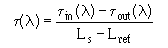Custom SearchChromatic Dispersion Chromatic, or intramodal, dispersion occurs in both single mode and multimode optical fibers. Chromatic dispersion occurs because different colors of light travel through the fiber at different speeds. Since the different colors of light have different velocities, some colors arrive at the fiber end before others. This delay difference is called the differential group delay τ(λ) per unit length. This differential group delay leads to pulse broadening. Chromatic dispersion is measured using EIA/TIA-455-168 in the time domain. Chromatic dispersion is also measured in the frequency domain using EIA/TIA-455-169 and EIA/TIA-455-175. These methods measure the composite optical fiber material and waveguide dispersion. To understand the contribution that material and waveguide dispersive mechanisms have on multimode and single mode fiber dispersion, refer to chapter 2. In this chapter we limit the discussion on chromatic dispersion to the time domain method described in EIA/TIA-455-168. The chromatic dispersion of multimode graded-index and single mode fiber is obtained by measuring fiber group delays in the time domain. These measurements are made using multiwavelength sources or multiple sources of different wavelengths. A multiwavelength source could be a wavelength-selectable laser. The pulse delay for both a long test sample fiber and a short reference fiber are measured over a range of wavelengths. The pulse delay for the reference fiber as a function of wavelength is &tgr;in(λ). The pulse delay for the test fiber as a function of wavelength is &tgr; out(λ). The group delay &tgr;(λ). per unit length at each wavelength iswhere Ls is the test sample fiber length in kilometers (km) and Lref is the reference sample length in km. The fiber chromatic dispersion is defined as the derivative, or slope, of the fiber group delay curve with respect to wavelength. Generally, the group delay as a function of wavelength is fit to a simple mathematical function and the derivative calculated. The range of wavelengths over which meaningful data is obtained depends on the wavelength range of optical source(s) used. The zero-dispersion wavelength (λ0) and the zero-dispersion slope (S0) are determined from the chromatic dispersion curve. Q.12 Delay differences between the source wavelengths occur as the optical signal propagates along the fiber. What is this called? Q.13 What determines the range of wavelengths over which meaningful data is obtained for calculating the chromatic dispersion?Integrated Publishing, Inc. - A (SDVOSB) Service Disabled Veteran Owned Small Business